Ask your WordPress questions! Pay money and get answers fast!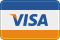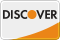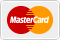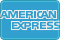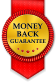# Check if two custom shortcodes are equal. WordPress

• SOLVED

I have two functions (one resides in functions.php<em>(sc2)</em> and the other is in a plugin<em>(sc1)</em>) I need one shortcode to check if the two are equal.

<strong>Sample Usage:</strong> [chapter_status id="664"]
This will check to see if the number of read pages equals the total number of subpages. I already have two functions I "just" need a shortcode that will check if the two are equal.

See functions below:
<em><strong>SC 1</strong></em>
```function dekciw_ru_count(\$atts){ global \$wpdb, \$post, \$current_user; get_currentuserinfo(); \$table_name = \$wpdb->prefix . "dekciw_ru"; extract(shortcode_atts(array('username' => '', 'parent' => ''), \$atts)); if(empty(\$username)) { \$username = \$current_user->user_login; } if(empty(\$parent)){ \$post_count = \$wpdb->get_var(\$wpdb->prepare("SELECT COUNT(*) FROM " . \$table_name . " WHERE author_name = '\$username' AND readstatus = '1';")); return \$post_count; } else { \$read_child_pages = 0; \$read_posts = \$wpdb->get_results("SELECT * FROM " . \$table_name . " WHERE author_name ='\$username' AND readstatus = '1';"); if(empty(\$read_posts)) { return 0;} foreach(\$read_posts as \$r_post){ \$read_posts_ids[] .= \$r_post->post_id; } \$children = get_posts(array('post_type' => 'page', 'post_parent' => \$parent, 'numberposts' => -1, 'post_status' => 'published')); foreach(\$children as \$child) { if(in_array(\$child->ID, \$read_posts_ids)) { \$read_child_pages++; } } return \$read_child_pages; /*print count(\$children);*/ } } add_shortcode('read_count', 'dekciw_ru_count'); ```

and
<em><strong>SC 2</strong></em>
```function sum_subpages(\$atts) { \$atts = extract(shortcode_atts(array('id'=>''),\$atts)); \$subpages = get_pages(array('child_of' => \$id, 'parent' => \$id, 'hierarchical' => 0)); \$amount = count(\$subpages); return \$amount; } add_shortcode('subpages','sum_subpages');```

So I need a function/shortcode that would basically do:

```if ([read_count parent=664]==[subpages id=664]) echo "<p>finished</p>"; else echo "<p>not finished</p>";```

Note: If it's needed or makes it easier I can move/copy the second function into the plugin file while there first one is since I cant move the first function as it uses a few variables it references from above.

Thanks,

2011-01-17

```if ( do_shortcode('[read_count parent=664]') == do_shortcode('[subpages id=664]')) echo "<p>finished</p>"; else echo "<p>not finished</p>";```

Thanks but this is not what I am looking for. This is just a template tag..
I need a shortcode where I can change the attribute and use in the loop.

```add_shortcode('chapter_status', 'chapter_status'); function chapter_status(\$atts) { if(!isset(\$atts['id'])) return; \$id = \$atts['id']; if(dekciw_ru_count(array('parent' => \$id)) == sum_subpages(array('id' => \$id))) { return "<p>Finished</p>"; } else { return "<p>Not Finished</p>"; } }```